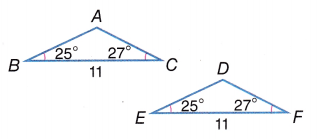# McGraw Hill Math Grade 8 Lesson 20.2 Answer Key Triangles: Congruent and Similar

Practice the questions of McGraw Hill Math Grade 8 Answer Key PDF Lesson 20.2 Triangles: Congruent and Similar to secure good marks & knowledge in the exams.

## McGraw-Hill Math Grade 8 Answer Key Lesson 20.2 Triangles: Congruent and Similar

Exercises

IDENTIFY

Identify each set of triangles as similar, congruent, or neither. Explain your answer.

Question 1.Congruent Triangles,

Explanation:
Two triangles are said to be congruent if pairs of their corresponding sides and their corresponding angles are equal.  Given triangles have equal sides and equal angles
therefore they are congruent.

Question 2.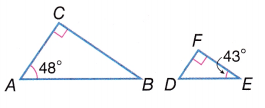Neither,

Explanation:
Given triangles have one common angle and lengths but they have different angles so they are neither congruent nor all similar angles.

Question 3.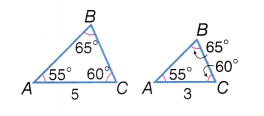Congruent Triangles,

Explanation:
Two triangles are said to be congruent if pairs of their corresponding sides and their corresponding angles are equal.  Given triangles have equal sides and equal angles
therefore they are congruent.

Question 4.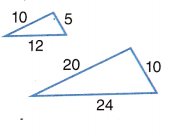Similar Triangles,

Explanation:
Similar triangles are triangles with same shape but with different sizes and also with same
angles. So given triangles are similar.

Question 5.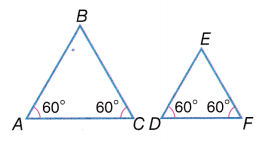Similar Triangles,

Explanation:
Similar triangles are triangles with same shape but with different sizes and also with same
angles. So given triangles are similar.

Question 6.